# Air Resistance Formula

Air Resistance Formula

Air resistance is a force that affects objects that move through the air. Often physics problems used in teaching ignore it, but it is very important for understanding the motion of fast-moving objects like airplanes. It depends on the density of the air, the area of the object, the velocity it is moving, and a "drag coefficient" that accounts for other properties of the object like the surface roughness, and turbulence. Air resistance is also called "drag", and the unit for this force is Newtons (N).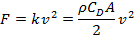F = force due to air resistance, or drag (N)

k = a constant that collects the effects of density, drag, and area (kg/m)

v = the velocity of the moving object (m/s)

ρ = the density of the air the object moves through (kg/m3)

CD = the drag coefficient, includes hard-to-measure effects (unitless)

A = the area of the object the air presses on (m2)

Air Resistance Formula Questions:

1) A large passenger jet is flying at a velocity of 250.0 m/s. The area of the airplane's wings facing the wind is A = 500.0 m2. The drag coefficient is CD = 0.024. At the altitude the airplane is flying, the density of the air is ρ = 0.4500 kg/m3. What is the force of air resistance acting on the passenger jet?

Answer: The force of air resistance can be found using the formula: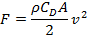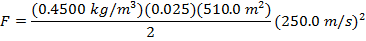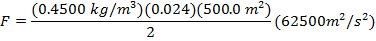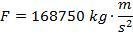F = 168750 N

The force of air resistance acting on the passenger jet is 168750 N.

2) A woman is floating toward the ground using a parachute. She is moving downward at a constant velocity, because the force upward from air resistance is balanced with the downward force of gravity. The force of gravity acting on her is 500 N. The density of the air at her current altitude is 1.20kg/m3, the area of the parachute is 75.0 m2, and the drag coefficient of the parachute is CD = 1.75. What is her downward velocity?

Answer: The parachutist's velocity can be found by rearranging the air resistance formula: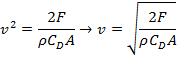She has a constant velocity, and so the force of gravity acting down must have the same value as the force of air resistance acting up. Therefore, the force F in the formula is equal to 500 N. Her downward velocity is: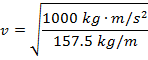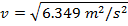v = 2.52 m/s

The downward velocity of the parachutist is 2.52 m/s.